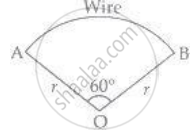# A piece of wire 20 cm long is bent into the form of an arc of a circle subtending an angle of 60° at its centre. Find the radius of the circle. - Mathematics

Sum

A piece of wire 20 cm long is bent into the form of an arc of a circle subtending an angle of 60° at its centre. Find the radius of the circle.

#### Solution

Arc is a part of circle that makes 60° between radii at endpoints A and B of wire.

So, it form the shape of a sector.r = ? l = 20 cm

∴ Length of arc l = (2 pir theta)/360^circ

⇒ 20 cm = (2 xx pi xx r xx 60^circ)/360^circ

⇒ 2pir = 20 xx 6

⇒ r = 120/(2pi) = 60/pi  cm

Concept: Area of Circle
Is there an error in this question or solution?

#### APPEARS IN

NCERT Mathematics Exemplar Class 10
Chapter 11 Area Related To Circles
Exercise 11.3 | Q 16 | Page 128

Share